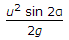# Civil Engineering - Applied Mechanics

### Exercise :: Applied Mechanics - Section 4

31.

A weight of 100 kg is supported by a string whose ends are attached to pegs A and B at the same level shown in below figure. The tension in the string is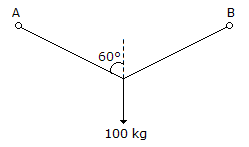A. 50 kg B. 75 kg C. 100 kg D. 120 kg.

Explanation:

No answer description available for this question. Let us discuss.

32.

A train weighing 196 tonnes experiences a frictional resistance of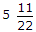per tonne. The speed of the train at the top of a down gradient 1 in 78.4 is 36 km/hour. The speed of the train after running 1 km down the slope, is

 A. 510 m/sec B. 105 m/sec C. 53 m/sec D. 35 m/sec.

Explanation:

No answer description available for this question. Let us discuss.

33.

The reaction at the support B of the beam shown in below figure is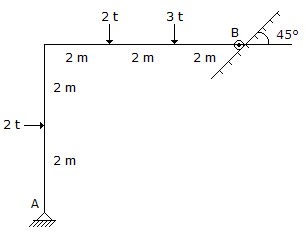A. 1.6 t B. 9.6 t C. 8.5 t D. 0.5 t.

Explanation:

No answer description available for this question. Let us discuss.

34.

A stone is whirled in a vertical circle, the tension in the string, is maximum

 A. when the string is horizontal B. when the stone is at the highest position C. when the stone is at the lowest position D. at all the positions.

Explanation:

No answer description available for this question. Let us discuss.

35.

If the velocity of projection is 4 m/sec and the angle of projection is α°, the maximum height of the projectile from a horizontal plane, is

 A.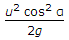B.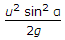C.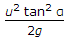D.